## Passive Control with Communication Delays

This example shows how to mitigate communication delays in a passive control system.

### Passivity-Based Control

By the Passivity Theorem, the negative-feedback interconnection of two strictly passive systemsandis always stable.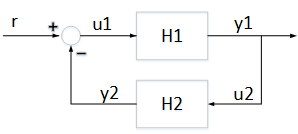When the physical plant is passive, it is therefore advantageous to use a passive controller for robustness and safety reasons. In networked control systems, however, communication delays can undo the benefits of passivity-based control and lead to instability. To illustrate this point, we use the plant and 2nd-order passive controller from the "Vibration Control in Flexible Beam" example. See this example for background on the underlying control problem. Load the plant modeland passive controller(note thatcorresponds toin the other example).

```load BeamControl G C bode(G,C,{1e-2,1e4}) legend('G','C') ```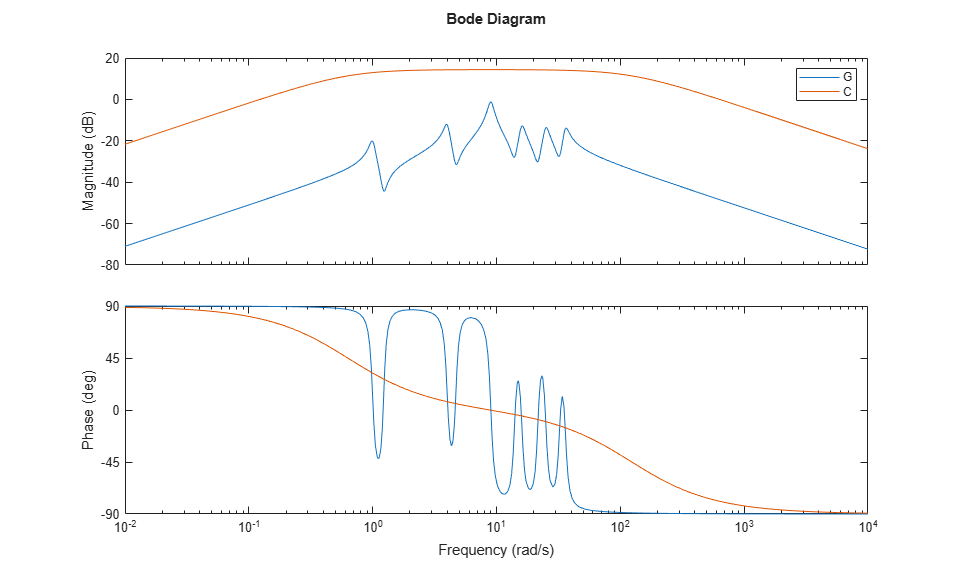The control configuration is shown below as well as the impulse response fromto.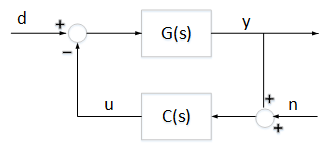```impulse(feedback(G,C)) ```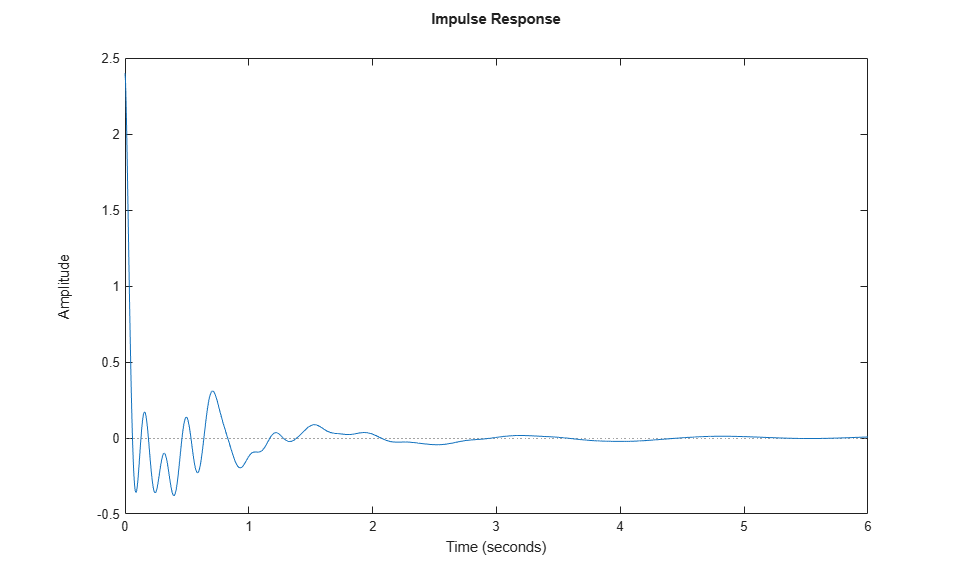### Destabilizing Effect of Communication Delays

Now suppose there are substantial communication delays between the sensor and the controller, and between the controller and the actuator. This situation is modeled in Simulink as follows.

```open_system('DelayedFeedback') ```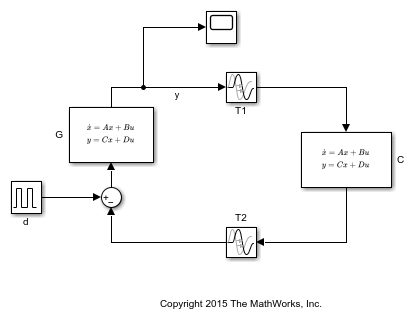The communication delays are set to

```T1 = 1; T2 = 2; ```

Simulating this model shows that the communication delays destabilize the feedback loop.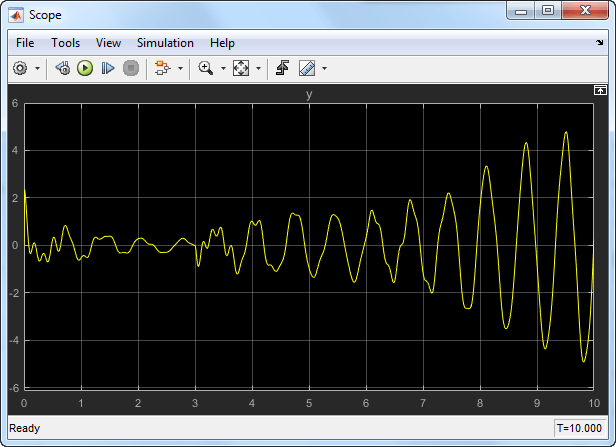### Scattering Transformation

To mitigate the delay effects, you can use a simple linear transformation of the signals exchanged between the plant and controller over the network.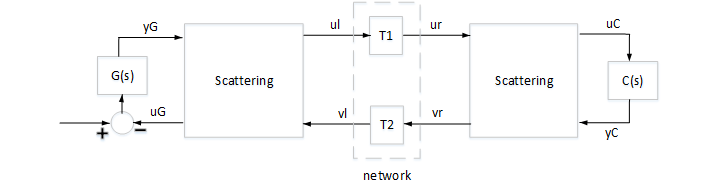Figure 1: Networked Control System

This is called the "scattering transformation" and given by the formulas

``

or equivalently

``

with. Note that in the absence of delays, the two scattering transformations cancel each other and the block diagram in Figure 1 is equivalent to the negative feedback interconnection ofand.

When delays are present, however,is no longer equal toand this scattering transformation alters the properties of the closed-loop system. In fact, observing that

``

and thatandstrictly passive ensures that

``

the Small Gain Theorem guarantees that the feedback interconnection of Figure 1 is always stable no matter how large the delays. Confirm this by building a Simulink model of the block diagram in Figure 1 for the value.

```b = 1; open_system('ScatteringTransformation') ```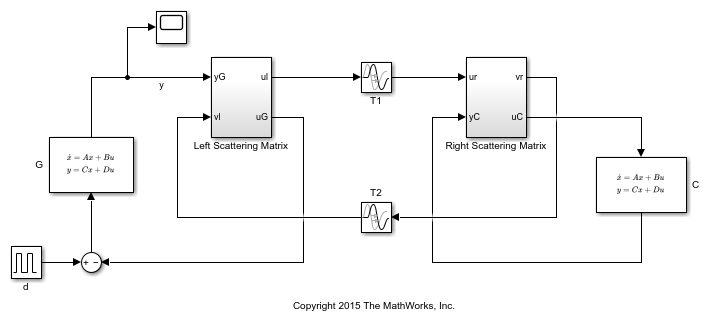Simulate the impulse response of the closed-loop system as done before. The response is now stable and similar to the delay-free response in spite of the large delays.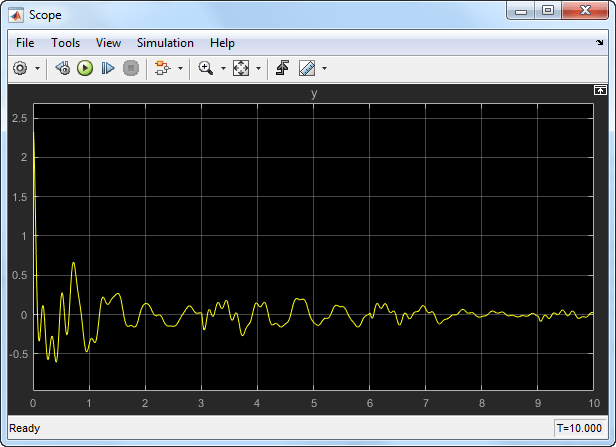For more details on the scattering transformation, see T. Matiakis, S. Hirche, and M. Buss, "Independent-of-Delay Stability of Nonlinear Networked Control Systems by Scattering Transformation," Proceedings of the 2006 American Control Conference, 2006, pp. 2801-2806.